# Math Practice Topic: Triangle Angles

 Description: Utilize the fact that the sum of angles in a triangle is 180. You may need pencil and paper. Adaptive Learning Progression: The number of problems increases. Start using MathScore for free

## Sample Levels (out of 6)

 1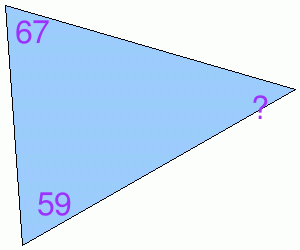? =

 1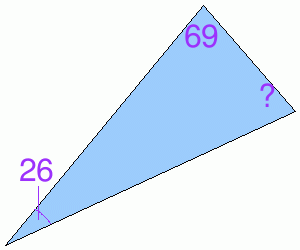? = 2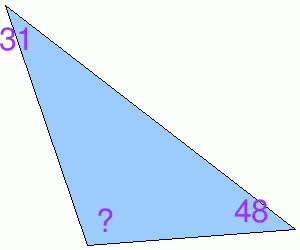? = 3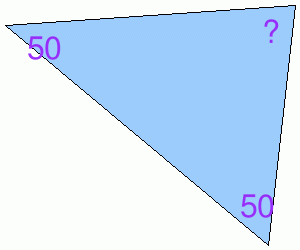? = 4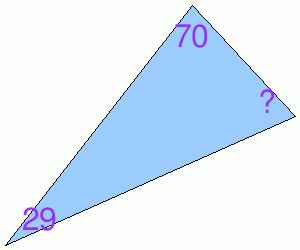? =